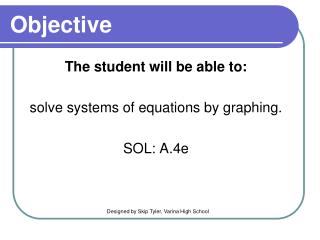DownloadDownload PresentationObjective

# Objective

Télécharger la présentation## Objective

- - - - - - - - - - - - - - - - - - - - - - - - - - - E N D - - - - - - - - - - - - - - - - - - - - - - - - - - -
##### Presentation Transcript

1. Objective The student will be able to: solve systems of equations by graphing. SOL: A.4e Designed by Skip Tyler, Varina High School

2. What is a system of equations? • A system of equations is when you have two or more equations using the same variables. • The solution to the system is the point that satisfies ALL of the equations. This point will be an ordered pair. • When graphing, you will encounter three possibilities.

3. Intersecting Lines • The point where the lines intersect is your solution. • The solution of this graph is (1, 2) (1,2)

4. Parallel Lines • These lines never intersect! • Since the lines never cross, there is NO SOLUTION! • Parallel lines have the same slope with different y-intercepts.

5. Coinciding Lines • These lines are the same! • Since the lines are on top of each other, there are INFINITELY MANY SOLUTIONS! • Coinciding lines have the same slope and y-intercepts.

6. What is the solution of the system graphed below? • (2, -2) • (-2, 2) • No solution • Infinitely many solutions

7. 1) Find the solution to the following system: 2x + y = 4 x - y = 2 Graph both equations. I will graph using x- and y-intercepts (plug in zeros). Graph the ordered pairs. 2x + y = 4 (0, 4) and (2, 0) x – y = 2 (0, -2) and (2, 0)

8. Graph the equations. 2x + y = 4 (0, 4) and (2, 0) x - y = 2 (0, -2) and (2, 0) Where do the lines intersect? (2, 0) 2x + y = 4 x – y = 2

9. Check your answer! To check your answer, plug the point back into both equations. 2x + y = 4 2(2) + (0) = 4 x - y = 2 (2) – (0) = 2 Nice job…let’s try another!

10. 2) Find the solution to the following system: y = 2x – 3 -2x + y = 1 Graph both equations. Put both equations in slope-intercept or standard form. I’ll do slope-intercept form on this one! y = 2x – 3 y = 2x + 1 Graph using slope and y-intercept

11. Graph the equations. y = 2x – 3 m = 2 and b = -3 y = 2x + 1 m = 2 and b = 1 Where do the lines intersect? No solution! Notice that the slopes are the same with different y-intercepts. If you recognize this early, you don’t have to graph them!

12. Check your answer! Not a lot to check…Just make sure you set up your equations correctly. I double-checked it and I did it right…

13. What is the solution of this system? • (3, 1) • (4, 4) • No solution • Infinitely many solutions 3x – y = 8 2y = 6x -16

14. Solving a system of equations by graphing. Let's summarize! There are 3 steps to solving a system using a graph. Graph using slope and y – intercept or x- and y-intercepts. Be sure to use a ruler and graph paper! Step 1: Graph both equations. This is the solution! LABEL the solution! Step 2: Do the graphs intersect? Substitute the x and y values into both equations to verify the point is a solution to both equations. Step 3: Check your solution.